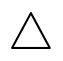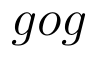Select Page

# Inverse Trigonometric Functions MCQ CBSE Maths 12 Science Solutions in English

Inverse Trigonometric Functions MCQ CBSE Maths 12 Science Solutions in English to enable students to get Solutions in a narrative video format for the specific question.

Expert Teacher provides Inverse Trigonometric Functions MCQ CBSE Maths 12 Science Solutions through Video Solutions in English language. This video solution will be useful for students to understand how to write an answer in exam in order to score more marks. This teacher uses a narrative style for a question from Inverse Trigonometric Functions not only to explain the proper method of answering question, but deriving right answer too.

Please find the question below and view the Solution in a narrative video format.

Question:

Solution Video in English:

You can select video Solutions from other languages also. Please check Solutions in ( Hindi )

## Similar Questions from CBSE, 12th Science, Maths, Inverse Trigonometric Functions

Question 1 : Using principal values, write the value of(View Answer Video)

Question 2 : Solve for(View Answer Video)

Question 3 : Evaluate :(View Answer Video)

Question 4 : Write the value of(View Answer Video)

Question 5 : Find the greatest and least values of(View Answer Video)

### Determinant

Question 1 : If=andis Cofactor of, then the value ofis given by (View Answer Video)

Question 2 : The solution of the following system of equation is 2x + 3y = 5, 5x – 2y = 3. (View Answer Video)

Question 3 : Evaluate. (View Answer Video)

Question 4 : Find the values of x, if. (View Answer Video)

Question 5 : If x, y, z are non-zero real numbers, then the inverse of matrixis, (View Answer Video)

### Relations and Functions

Question 1 : Number of binary sets on the setis, (View Answer Video)

Question 2 : Functionsare defined respectively, by, find(View Answer Video)

Question 3 : Let A = {1, 2, 3, 4} and let R = {(2, 2), (3, 3), (4, 4), (1, 2)} be a relation on A. Then, R is, (View Answer Video)

Question 4 :  If f(x) = ax + b and g(x) = cx + d, then f[g(x)] – g[f(x)] is equivalent to, (View Answer Video)

Question 5 : Let * be the binary operation on N given by a * b = LCM of a and b. Find the identity of * in N? (View Answer Video)

### Application of Derivatives

Question 1 : The line y = mx + 1 is a tangent to the curveif the value of m is: (View Answer Video)

Question 2 : The point on the curvewhich is nearest to the point (0, 5) is :
Question 4 : Find the maximum profit that a company can make if the profit function is given by(View Answer Video)
Question 5 : Sand is pouring from a pipe at the rate of. The falling sand forms a cone on the ground in such a way that the height of the cone is always one-sixth of the radius of the base. How fast is the height of the sand cone increasing, when the height is 4 cm? (View Answer Video)